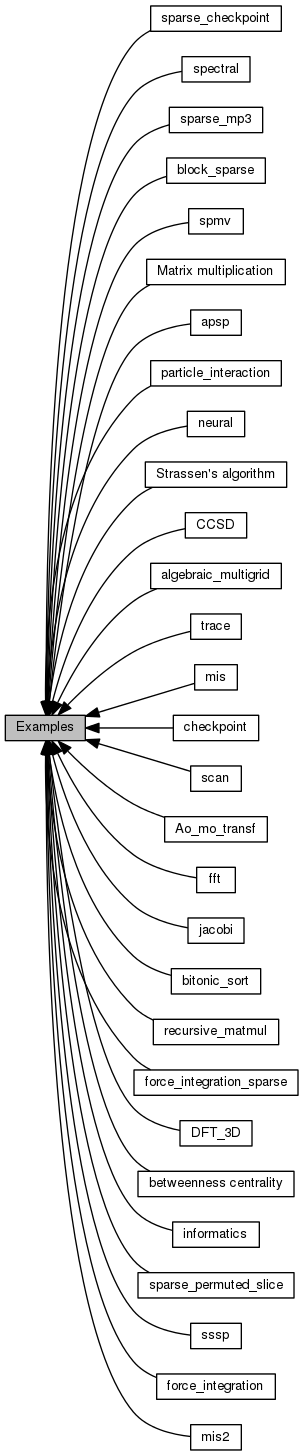Cyclops Tensor Framework parallel arithmetic on multidimensional arrays
Examples
Collaboration diagram for Examples:## Modules

algebraic_multigrid
Benchmark for smoothed algebraic multgrid.

Ao_mo_transf
Transformation between atomic and molecular orbitals.

apsp
All-pairs shortest-paths via path doubling and Tiskin's augmented path doubling.

bitonic_sort
bitonic_sort sort iterative method using gemv and spmv

block_sparse
All-pairs shortest-paths via path doubling and Tiskin's augmented path doubling.

betweenness centrality
betweenness centrality computation

CCSD
A Coupled Cluster Singles and Doubles contraction code extracted from Aquarius.

checkpoint
tests read and write dense data to file functionality

sparse_checkpoint
tests read and write dense data to file functionality

DFT_3D
3D Discrete Fourier Transform by tensor contractions

fft
FFT iterative method using gemv and spmv.

force_integration
tests custom element-wise transform by doing force integration

force_integration_sparse
tests custom element-wise transform on sparse tensors by doing force integration

jacobi
Jacobi iterative method using gemv and spmv.

Matrix multiplication
Multiplication of two matrices with user-defined attributes of symmetry and sparsity.

mis
code for maximal independent set

mis2
code for maximal 2-independent set

neural
Neural Network.

particle_interaction
tests custom element-wise functions by computing interactions between particles and integrating

recursive_matmul
Performs recursive parallel matrix multiplication using the slice interface to extract blocks.

scan
scan iterative method using gemv and spmv

sparse_mp3
Third-order Moller-Plesset petrubation theory (MP3) with sparse integrals. Equations adapted from those in Aquarius (credit to Devin Matthews)

sparse_permuted_slice
Randomly permuted block write of symmetric matrices from matrix on COMM_SELF to symmetric matrix on COMM_WORLD.

spectral
Spectral element methods test/benchmark.

spmv
Multiplication of a random square sparse matrix by a vector.

sssp
single-source shortest-paths via the Bellman-Ford algorithm

Strassen's algorithm
Strassen's algorithm using the slice interface to extract blocks.

trace
tests trace over diagonal of Matrices

informatics
high-order nonsymmetric contractions